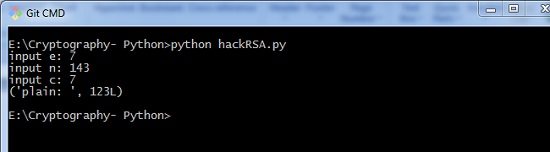# Hacking RSA Cipher

Hacking RSA cipher is possible with small prime numbers, but it is considered impossible if it is used with large numbers. The reasons which specify why it is difficult to hack RSA cipher are as follows −

• Brute force attack would not work as there are too many possible keys to work through. Also, this consumes a lot of time.

• Dictionary attack will not work in RSA algorithm as the keys are numeric and does not include any characters in it.

• Frequency analysis of the characters is very difficult to follow as a single encrypted block represents various characters.

• There are no specific mathematical tricks to hack RSA cipher.

The RSA decryption equation is −

M = C^d mod n


With the help of small prime numbers, we can try hacking RSA cipher and the sample code for the same is mentioned below −

def p_and_q(n):
data = []
for i in range(2, n):
if n % i == 0:
data.append(i)
return tuple(data)

def euler(p, q):
return (p - 1) * (q - 1)

def private_index(e, euler_v):
for i in range(2, euler_v):
if i * e % euler_v == 1:
return i

def decipher(d, n, c):
return c ** d % n
def main():
e = int(input("input e: "))
n = int(input("input n: "))
c = int(input("input c: "))

# t = 123
# private key = (103, 143)
p_and_q_v = p_and_q(n)
# print("[p_and_q]: ", p_and_q_v)
euler_v = euler(p_and_q_v, p_and_q_v)

# print("[euler]: ", euler_v)
d = private_index(e, euler_v)
plain = decipher(d, n, c)
print("plain: ", plain)
if __name__ == "__main__":
main()


## Output

The above code produces the following output −